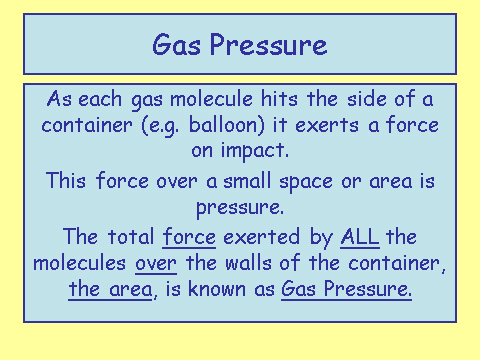# Particle Motion in Gases

## Motion of Particles in a Gas

The motion of particles in a gas is constant __and __random. This means that all of the particles are a__lways moving__ and that there is __no pattern __to their movement.

Temperature of gas is related to the average kinetic energy of the particles/molecules. Earlier we described temperature as how much a particle moves, the greater the temperature the greater the kinetic energy. We can see that this description is useful here as well.

For a given gas at a constant volume (so its container does not change), a changing temperature __can change the __pressure.

## How Does Temperature and Pressure Affect the Motion of a Particle?With gas pressure defined we can look at how the motion of a particle changes with temperature and pressure.

At a constant volume if the temperature increases, so does the __pressure __and vice versa.

This means that if the molecules/particles are under greater pressure then the temperature will rise and the motion will increase. The particles will move more and faster.

Likewise, if the temperature increases, the pressure will increase and the particles will move more and faster.

﻿

## Absolute Zero

Absolute zero is found at around -273 degrees C. At this point the the particles in a substance have no energy. This means that there is no movement at all!

In reality it is actually impossible to get to absolute zero but we are able to cool substances down to temperatures very close to this value.

The scientific temperature scale uses a different scale called Kelvin. Absolute zero is 0 degrees Kelvin or 0K (That’s a zero and a capital ‘K’). Kelvin has the same interval as celsius so a degree step up in Kelvin is the same amount as celsius. This leads to an easy conversion as we just need to add the number 273 when converting from celsius to Kelvin. We minus 273 when going from Kelvin to celsius.

Interestingly space is around 2K, the coldest on Earth ever (2017) was recorded in a lab which was a frosty 0.00036K

If the temperature is increased, what happens to the motion of the particles of a gas if the volume is kept the same?
increases
If the temperature is increased, what happens to the pressure of a gas if the volume is kept the same?
increases
Describe the motion of gas particles?
random
Convert 298K into celsius
25
Explanation: 25 degrees C
Convert 100 degrees C into Kelvin
373K